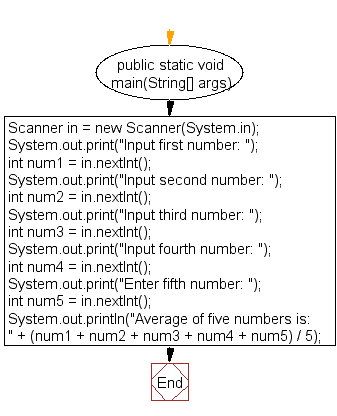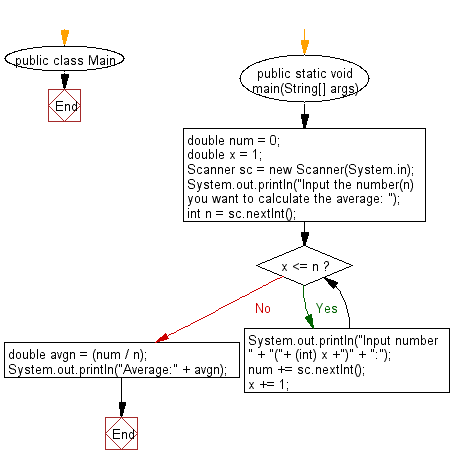﻿ Java: Calculate and print the average of five numbers# Java Exercises: Calculate and print the average of five numbers

## Java Basic: Exercise-12 with Solution

Write a Java program that takes five numbers as input to calculate and print the average of the numbers.

Test Data:
Input first number: 10
Input second number: 20
Input third number: 30
Input fourth number: 40
Enter fifth number: 50

Pictorial Presentation:Sample Solution:

Java Code:

``````import java.util.Scanner;

public class Exercise12 {

public static void main(String[] args) {
Scanner in = new Scanner(System.in);

System.out.print("Input first number: ");
int num1 = in.nextInt();

System.out.print("Input second number: ");
int num2 = in.nextInt();

System.out.print("Input third number: ");
int num3 = in.nextInt();

System.out.print("Input fourth number: ");
int num4 = in.nextInt();

System.out.print("Enter fifth number: ");
int num5 = in.nextInt();

System.out.println("Average of five numbers is: " +
(num1 + num2 + num3 + num4 + num5) / 5);
}
}
```
```

Sample Output:

```Input first number: 10
Input second number: 20
Input third number: 30
Input fourth number: 40
Enter fifth number: 50
Average of five numbers is: 30
```

Flowchart:Sample Solution:

Java Code:

``````import java.util.Scanner;

public class Main {
public static void main(String[] args) {
double num = 0;
double x = 1;
Scanner sc = new Scanner(System.in);
System.out.println("Input the number(n) you want to calculate the average: ");
int n = sc.nextInt();
while (x <= n) {
System.out.println("Input number " + "("+ (int) x +")" + ":");
num += sc.nextInt();
x += 1;
}
double avgn = (num / n);

System.out.println("Average:" + avgn);
}
}
```
```

Sample Output:

```Input the number(n) you want to calculate the average:
4
Input number (1):
2
Input number (2):
4
Input number (3):
4
Input number (4):
2
Average:3.0
```

Flowchart:Java Code Editor:

What is the difficulty level of this exercise?

Test your Programming skills with w3resource's quiz.

﻿

## Java: Tips of the Day

countOccurrences

Counts the occurrences of a value in an array.

Use Arrays.stream().filter().count() to count total number of values that equals the specified value.

```public static long countOccurrences(int[] numbers, int value) {
return Arrays.stream(numbers)
.filter(number -> number == value)
.count();
}
```

Ref: https://bit.ly/3kCAgLb